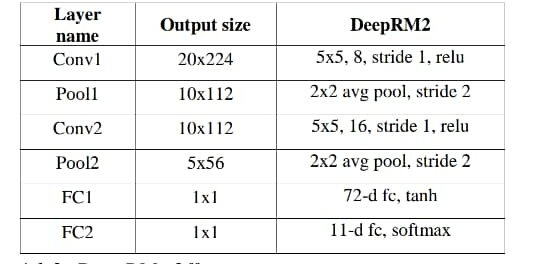# 4D tensor equivalent neural network layer in PyTorch

Hello,
How can I define a layer like below code in pytorch?

``````InputLayer(
shape=(None, 1, input_height, input_width),
)
``````

(The input is a 4 Dimensional tensor.)

there is no need to specify an input layer, you can directly start using convolutional or linear layers in pytorch via torch.nn.Conv or torch.nn.Linear.

If you have any more questions, just post them.

@ SiQube I have below neural network in Theano library, and want to find its equivalent neural network in PyTorch.

``````l_in = lasagne.layers.InputLayer(
shape=(None, 1, input_height, input_width),
)

l_hid = lasagne.layers.DenseLayer(
l_in,
num_units=20,
# nonlinearity=lasagne.nonlinearities.tanh,
nonlinearity=lasagne.nonlinearities.rectify,
# W=lasagne.init.Normal(.0201),
W=lasagne.init.Normal(.01),
b=lasagne.init.Constant(0)
)

l_out = lasagne.layers.DenseLayer(
l_hid,
num_units=output_length,
nonlinearity=lasagne.nonlinearities.softmax,
# W=lasagne.init.Normal(.0001),
W=lasagne.init.Normal(.01),
b=lasagne.init.Constant(0)
)
``````

I just want to know how covet l_in layer to PyTorch layer and pass it to L-hid.
I wrote below code which is not completed but it could clarify what I need.

`````` def __init__(self, input_height, input_width, output_length):
super(Net, self).__init__()

**self.fc1 = nn.Linear(input_height * input_width, 20)**  (this  layer must be change and become sutable for 4D tensor)
self.fc1.weight.data.fill_(torch.nn.init.normal_(.01))
self.fc1.bias.data.fill_(torch.nn.init.constant(0))
self.fc2 = nn.Linear(20, output_length)
self.fc2.weight.data.fill_(torch.nn.init.normal_(.01))
self.fc2.bias.data.fill_(torch.nn.init.constant(0))

def forward(self, x):
x = F.relu(self.fc1(x))
x = F.softmax(self.fc2(x))
return x
``````

The code for your layers looks fine. There are multiple ways in PyTorch for flattening a multi-dimensional input. In your case, one option is to change your `forward` method to something like

``````def forward(self, x):
x = torch.flatten(x, start_dim=1)  # Assumes input 'x' is batched
x = F.relu(self.fc1(x))
x = F.softmax(self.fc2(x))
return x
``````

Note that you could also combine the flatten instruction with the first line in your example;

``````def forward(self, x):
x = F.relu(self.fc1(x.flatten(start_dim=1))  # Assumes input 'x' is batched
x = F.softmax(self.fc2(x))
return x
``````

Personally, I prefer the first alternative since I find it more readable.

UPDATE: You could also solve this by using `torch.nn.Flatten`.

Oh, that you can in the forward pass, you reshape your tensor via

``````def forward(self, x):
x = x.view(batch_size, -1)
x = F.relu(self.fc(x))
``````

Thank you for your attention. Now I don’t want to flatting my input. My input is (10, 1, 20, 224). I want to pass this tensor to l_in but I don’t know how pass it to first layer of my network and how pass result of this layer to fc2.
Simply I want equivalent of `l_in = lasagne.layers.InputLayer( shape=(None, 1, input_height, input_width), )` in constructing my neural network with (10, 1, 20, 224) tensor.

If you look at the documentation for `lasagna.layers.DenseLayer`, you will see that they implictly perform a flattening operation for you whenever the input has more than two axes. In other words, you have been using flattened inputs in Theano, but it was “automagically” performed1 Like

So thomasjo

``````self.fc1 = nn.Linear(input_height * input_width, 20)
``````

must be change to

``````self.fc1 = nn.Linear(input, 20)
``````

which input is (10, 1, 20, 224) tensor and will be flatted by

``````x = torch.flatten(x, start_dim=1)  # Assumes input 'x' is batched
``````

Is it right?

I do not remember the details of Theano’s memory layout, but I am assuming it uses the NCHW format, which means your input dimensions `(10, 1, 20, 224)` corresponds to

• batch size of 10,
• channel depth of 1,
• image height of 20 pixels,
• image width of 224 pixels.

(The image height of 20 pixels does seem a bit odd to me – 224 would be more typical – but I am assuming it is correct. Change the examples below if it is incorrect.)

Under these assumptions, you do not need to change your declaration of `fc1`. That layer expects an input tensor with dimensions `[None, 20 * 224]` and will output a tensor with dimensions `[None, 20]`.

The flattening operation will ensure that an input tensor with dimensions `[None, 1, 224, 20]` is transformed into a tensor with dimensions `[None, 1 * 20 * 224]`, which is exactly the same as layer `fc1` expects.

Note that if you switch from images with 1 channel to e.g. 3 channels (RGB), you will need to update your definition of `fc1` accordingly, but you will not need to change the `torch.flatten` operation.

Sir, thomasjo
If I want to use conventional layer to transfer (10, 1, 20, 244) tensor to 11 output like blow table. How should I change my neural network?This is sort of tangential to your original question, but something like this should work for defining the layers/modules

``````def __init__(self):
super().__init__()

self.conv1 = nn.Conv2d(in_channels=1, out_channels=8, kernel_size=(5, 5), stride=1)
self.pool1 = nn.AvgPool2d(kernel_size=(2, 2), stride=2)

self.conv2 = nn.Conv2d(in_channels=8, out_channels=16, kernel_size=(5, 5), stride=1)
self.pool2 = nn.AvgPool2d(kernel_size=(2, 2), stride=2)

self.fc1 = nn.Linear(in_features=16 * 5 * 56, out_features=72)
self.fc2 = nn.Linear(in_features=72, out_features=11)
``````

Then, in your `forward` method, you could do something like

``````def forward(self, x):
x = self.conv1(x)
x = nn.functional.relu(x, inplace=True)
x = self.pool1(x)

x = self.conv2(x)
x = nn.functional.relu(x, inplace=True)
x = self.pool2(x)

x = torch.flatten(x, start_dim=1)
x = self.fc1(x)
x = nn.functional.tanh(x)

x = self.fc2(x)
x = nn.functional.softmax(x)

return x
``````

Please, note that there exist alternative (and arguably better) ways of defining the network and the forward pass. I chose to present the examples this way to better match your table and hopefully be easier to understand. If you want to improve the code, I highly recommend using the `nn.Sequential` container module. This would allow you to greatly simplify the `forward` method by declaring each “convolutional block” as a single unit, including ReLU and average pooling. I will leave this as an exercise for you to figure out.

Also be aware that I have not tested any of the code, so it is very likely that I have made some mistakes.